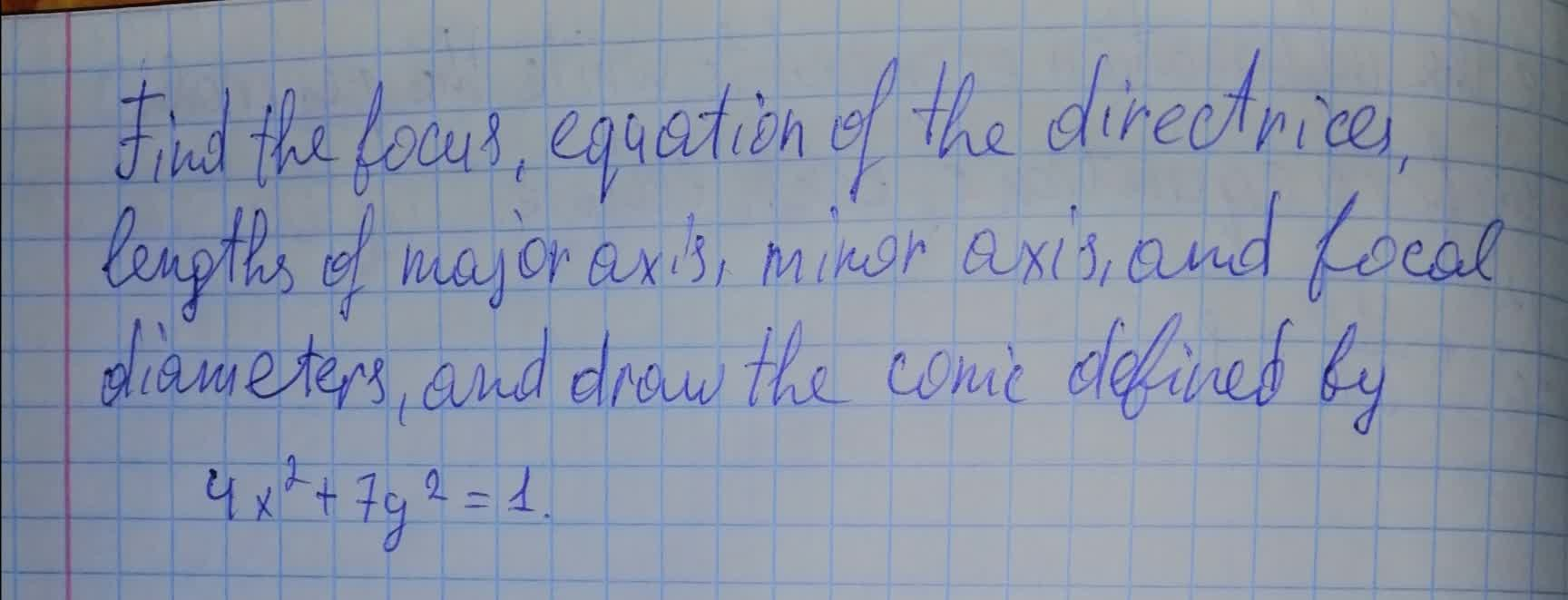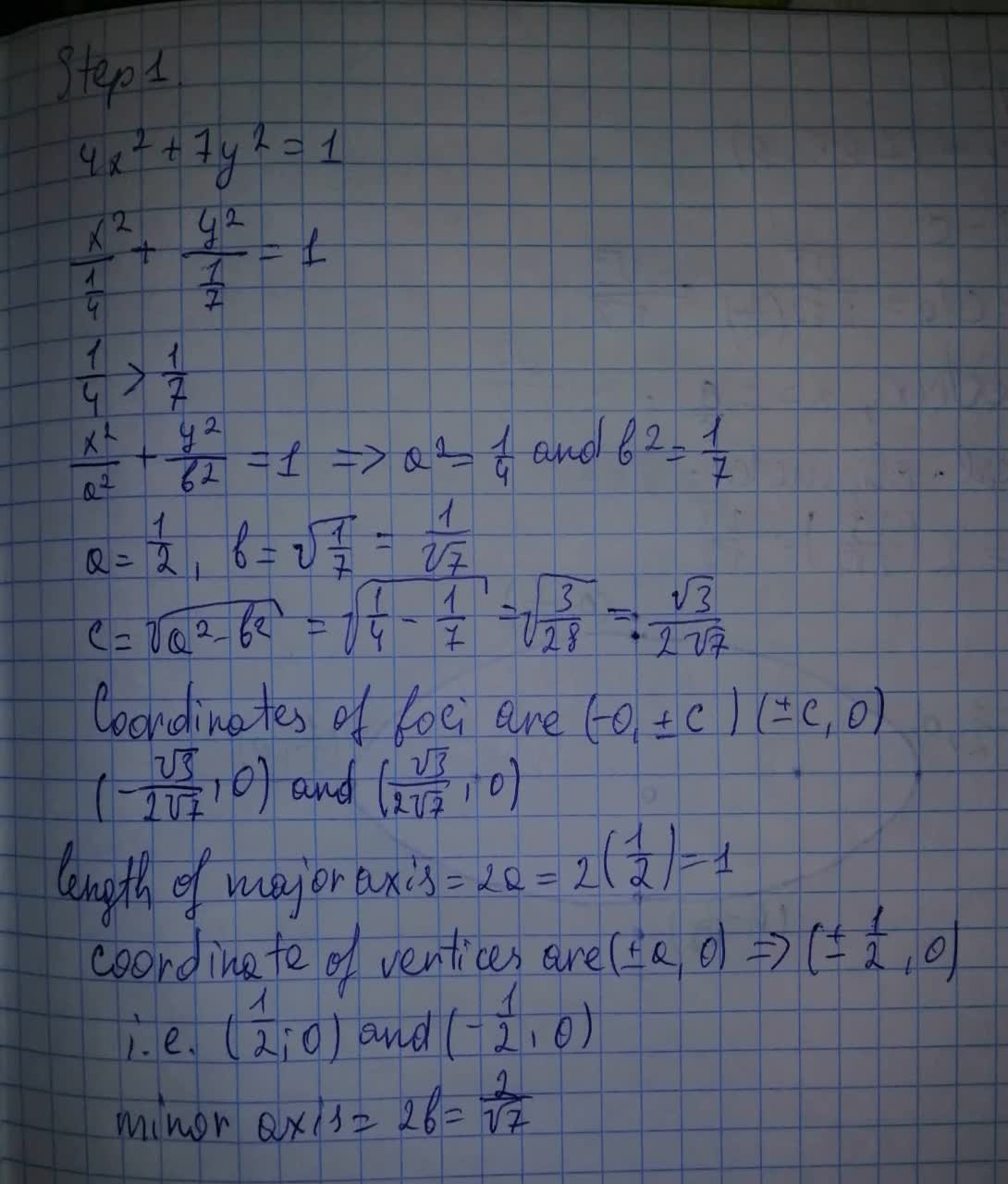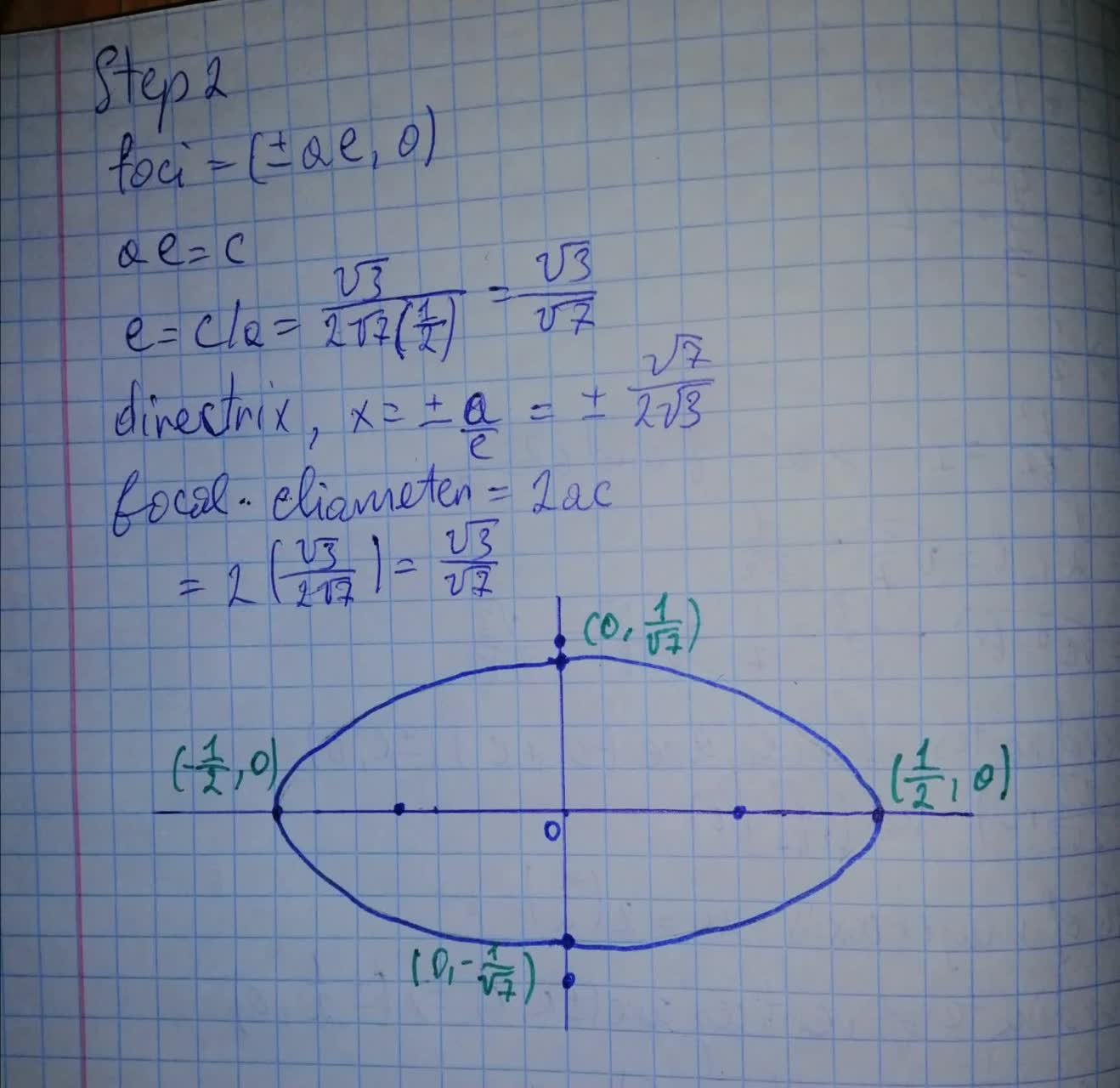Find the focus, equation of the directrices, lengths of major axis, minor axis and focal diameters, and draw the conic defined by 4x^2+7y^2=1chillywilly12a 2021-08-12 Answered
Find the focus, equation of the directrices, lengths of major axis, minor axis and focal diameters, and draw the conic defined by
$$\displaystyle{4}{x}^{{2}}+{7}{y}^{{2}}={1}$$• Questions are typically answered in as fast as 30 minutes

Solve your problem for the price of one coffee

• Math expert for every subject
• Pay only if we can solve itPohanginah

Calculation: